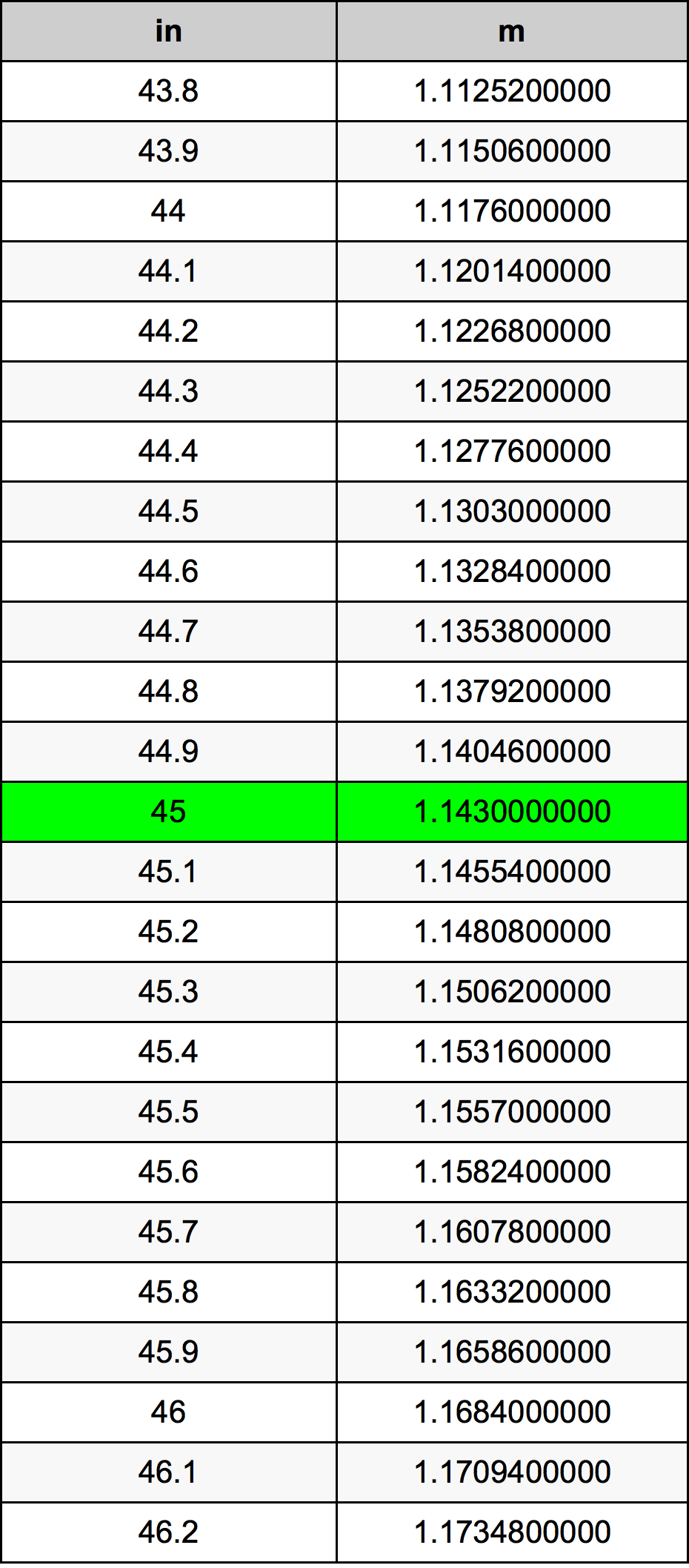Inches To Meters

# 45 in to m45 Inches to Meters

in
=
m

## How to convert 45 inches to meters?

 45 in * 0.0254 m = 1.143 m 1 in
A common question is How many inch in 45 meter? And the answer is 1771.65354331 in in 45 m. Likewise the question how many meter in 45 inch has the answer of 1.143 m in 45 in.

## How much are 45 inches in meters?

45 inches equal 1.143 meters (45in = 1.143m). Converting 45 in to m is easy. Simply use our calculator above, or apply the formula to change the length 45 in to m.

## Convert 45 in to common lengths

UnitUnit of length
Nanometer1143000000.0 nm
Micrometer1143000.0 µm
Millimeter1143.0 mm
Centimeter114.3 cm
Inch45.0 in
Foot3.75 ft
Yard1.25 yd
Meter1.143 m
Kilometer0.001143 km
Mile0.0007102273 mi
Nautical mile0.0006171706 nmi

## What is 45 inches in m?

To convert 45 in to m multiply the length in inches by 0.0254. The 45 in in m formula is [m] = 45 * 0.0254. Thus, for 45 inches in meter we get 1.143 m.

## 45 Inch Conversion Table## Alternative spelling

45 in to Meter, 45 in in Meter, 45 Inch to Meter, 45 Inch in Meter, 45 Inches to Meters, 45 Inches in Meters, 45 Inch to m, 45 Inch in m, 45 Inches to Meter, 45 Inches in Meter, 45 Inch to Meters, 45 Inch in Meters, 45 in to Meters, 45 in in Meters